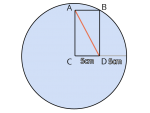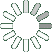Puzzle Command
Daily Puzzle
Diagonal Line
December 10, 2014As shown in the image, there is a circle.Inside circle the there is a rectangle with points ABCD. Assuming that point C is in the center of the circle. How long is the diagonal line AD?

Puzzle Notes
You don't need the Pythagorean Theorem to answer this question.
Enter in your solution below and click submit.